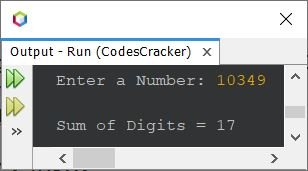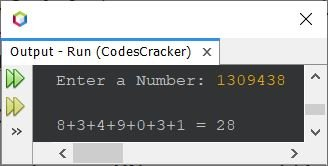# Java Program to Find Sum of Digits of a Number

This article covers a program in Java that find and prints the sum of all digits of a number entered by user. For example, if entered number is 13049, then the output will be 17. 17 is the sum of all digits of given number, i.e., 1+3+0+4+9.

## Find Sum of Digits in Java using while Loop

The question is, write a Java program to find the sum of digits of a given number using while loop. The program given below is its answer:

```import java.util.Scanner;

public class CodesCracker
{
public static void main(String[] args)
{
int num, digit, sum=0;
Scanner s = new Scanner(System.in);

System.out.print("Enter a Number: ");
num = s.nextInt();

while(num!=0)
{
digit = num%10;
sum = sum + digit;
num = num/10;
}

System.out.println("\nSum of Digits = " +sum);
}
}```

The snapshot given below shows the sample run produced by above Java program, with user input 10349 as number to find and print the sum of its all digits:That is, 1+0+3+4+9 is equal to 17. The same program can also be created in this way:

```import java.util.Scanner;

public class CodesCracker
{
public static void main(String[] args)
{
int num, digit, sum=0;
Scanner s = new Scanner(System.in);

System.out.print("Enter a Number: ");
num = s.nextInt();

System.out.print("\n");
while(num!=0)
{
digit = num%10;
sum = sum + digit;
num = num/10;
if(num!=0)
System.out.print(digit + "+");
else
System.out.print(digit+ " = " + sum);
}
}
}```

Here is its sample run with user input, 1309438:Also the while block can be written in this way too:

```while(true)
{
digit = num%10;
sum = sum + digit;
num = num/10;
if(num!=0)
System.out.print(digit + "+");
else
{
System.out.print(digit+ " = " + sum);
break;
}
}```

## Find Sum of Digits in Java using for Loop

Let's see how the same program looks like, when created using for loop.

```import java.util.Scanner;

public class CodesCracker
{
public static void main(String[] args)
{
int num, digit, sum;
Scanner s = new Scanner(System.in);

System.out.print("Enter a Number: ");
num = s.nextInt();

for(sum=0; num!=0; num = num/10)
{
digit = num%10;
sum = sum + digit;
}

System.out.println("\nSum of Digits = " +sum);
}
}```

## Find Sum of Digits using Function

This program is created using function. In this program, I've created a function named sumOfDigits() that takes a single argument and returns the sum of digits of that argument.

```import java.util.Scanner;

public class CodesCracker
{
public static void main(String[] args)
{
int num, digit, sum=0;
Scanner s = new Scanner(System.in);

System.out.print("Enter a Number: ");
num = s.nextInt();

System.out.println("\nSum of Digits = " +sumOfDigits(num));
}
public static int sumOfDigits(int n)
{
int sum = 0;
while(n!=0)
{
sum = sum + (n%10);
n /= 10;
}
return sum;
}
}```

#### Same Program in Other Languages

Java Online Test

« Previous Program Next Program »